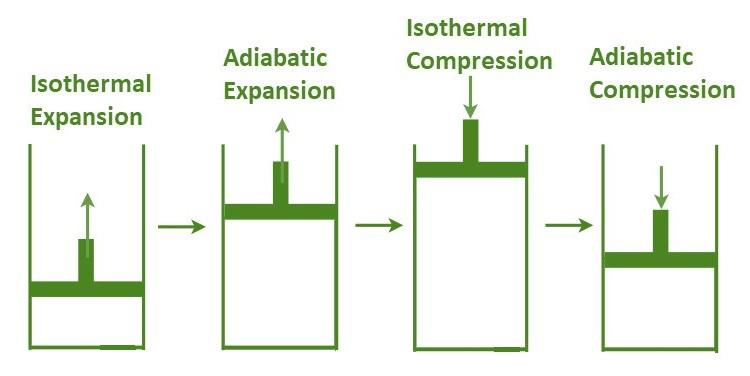GeeksforGeeks App
Open AppBrowser
Continue

## Related Articles

A Carnot motor is a hypothetical motor that works on the Carnot cycle. Nicolas Leonard Sadi Carnot fostered the fundamental model for this motor in 1824. In this unmistakable article, you will find out about the Carnot cycle and Carnot Theorem exhaustively. The Carnot motor is a hypothetical thermodynamic cycle proposed by Leonard Carnot.

## Carnot Engine Theory

Any framework working between T1 (hot supply) and T2 (cold repository) can never have more effectiveness than the Carnot motor working between similar repositories. It gauges the most extreme conceivable productivity that an intensity motor during the transformation interaction of intensity into work and, on the other hand, working between two supplies can have. Additionally, the productivity of this kind of motor is free of the idea of the functioning substance and is just subject to the temperature of the hot and cold supplies.

## Carnot Cycle

A Carnot cycle is characterized as an optimal reversible shut thermodynamic cycle. Four progressive activities are involved: isothermal development, adiabatic extension, isothermal pressure, and adiabatic pressure. During these activities, the extension and pressure of the substance should be possible up to the ideal point and back to the underlying state.Carnot Cycle

## Four Processes of the Carnot Cycle

1. In (a), the cycle is reversible isothermal gas development. In this cycle, how much intensity is consumed by the ideal gas is qin from the intensity source at a temperature of Th. The gas grows and takes care of business on the environmental factors.
2. In (b), the cycle is reversible adiabatic gas development. Here, the framework is thermally protected, the gas proceeds to grow, and work is finished on the environmental elements. Presently the temperature is lower, Tl.
3. In (c), the cycle is a reversible isothermal gas pressure process. Here, the intensity misfortune out happens when the environmental factors accomplish the work at temperature Tl.
4. In (d), the cycle is reversible adiabatic gas pressure. Again the framework is thermally protected. The temperature again increases back to Th as the encompassing keep on taking care of their responsibilities on the gas.

### Isothermal Development

The gas is taken from P1, V1, T1 to P2, V2, and T2. Heat Q1 is ingested from the repository at temperature T1. Since the development is isothermal, the all-out change in inward energy is zero, and the intensity consumed by the gas is equivalent to the work done by the gas on the climate, which is given as:

W1 ⇢ 2 = Q1 = μ × R × T1 × ln(υ2 ⁄ υ1)

In this expansion, the gas extends adiabatically from P2, V2, and T1 to P3, V3, and T2.

W2 ⇢ 3 = (μ R / γ-1) (T1 – T2)

### Isothermal Pressure

In this expansion, the gas is packed isothermally from the state (P3, V3, T2) to (P4, V4, T2).

W3 ⇢ 4 = μ R T2 ln (υ3 / υ4)

In this process, the gas is compacted adiabatically from the state (P4, V4, T2) to (P1, V1, T1).

W4 ⇢ 1 = (μ R / γ – 1) (T1 – T2)

The total outwork done by the gas on the climate in one complete cycle is given by,

W = W1⇢2 + W2⇢3 + W3⇢4 + W4⇢1

Net efficiency = Net work done by the gas/Heat absorbed by the gas

Net efficiency = (W/Q1) =(Q1 – Q2)/Q1) =1-(Q2/Q1)

Since stage 2->3 is an adiabatic interaction, we can compose, T1 V2Ƴ-1 = T2 V3Ƴ-1 or (υ2 / υ3) = (T2 / T1)1/γ-1.

Similarly, (υ1 / υ2) = (T2 / T1)1/γ-1

This implies, υ2 / υ3 = υ12

Thus, the articulation for net productivity of Carnot motor diminishes to the net efficiency of (1 – T2/T1).

## Solved Examples On Carnot Engine

Example 1. The intensity consumed by a motor is, Q1 = 8,000 Joule, T1 = 1600 K, and T2 = 800 K. What is the work done by the Carnot motor?

Solution:

Given:

Q1 = 8000

T1 = 1600

T2 = 800

The effectiveness of the Carnot motor,

e = (T1 – T2)/T1

= (1600 – 800)/1600

= 800/1600

= 1/2

Work done by Carnot motor is,

W = eQ1

= (1/2) × 8000

= 4000 J

Example 2. The intensity consumed by a motor is, Q1 = 1000 Joule, T1 = 700 K, and T2 = 250 K. What is the work done by the Carnot motor?

Solution:

Given:

Q1 = 1000

T1 = 700

T2 = 250

The effectiveness of the Carnot motor,

e = (T1 – T2)/T1

= (700 – 250)/700

= 450/700

= 0.64

Work done by Carnot motor is,

W = eQ1

= 0.64 × 8000

= 642.85 J

Example 3. The intensity consumed by a motor is, Q = 350 J. What is the work done by the Carnot motor if the effectiveness is 0.7?

Solution:

We have,

e = 0.7

Q = 350

Work done by Carnot motor is,

W = eQ

= 0.7 × 350

= 245 J

Example 4. The work done by the Carnot motor is 500 J. Find the intensity of the motor if the effectiveness is 0.45.

Solution:

We have,

W = 500

e = 0.45

Work done by Carnot motor is,

W = eQ

=> Q = W/e

= 500/0.45

= 1111.11 J

## FAQs On Carnot Engine

Question 1. Which gases satisfy the conditions of a Carnot Engine?

Solution:

Only ideal gases can fulfil the requirements of a Carnot engine as it is a theoretical engine.

Question 2. What is a reversible Carnot engine?

Solution:

The cycle defined as a Carnot heat-engine is completely reversible. It can become the Carnot heat pump and refrigeration cycle if all the operations that make it up are reversed.

Question 3. What are the various processes used in the Carnot engine?

Solution:

There are four processes involved in the Carnot engine. These are isothermal expansion, adiabatic expansion, isothermal compression and adiabatic compression.

My Personal Notes arrow_drop_up
Related Tutorials